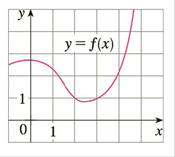Chapter 2.5, Problem 66E

Chapter
Section
Textbook Problem
3 views

# If f is the function whose graph is shown, let h ( x ) = f ( f ( x ) ) and g ( x ) = f ( x 2 ) . Use the graph of f to estimate the value of each derivative.(a) h′(2)(b) g′(2)To determine

(a)

To find:

h '(2)

Explanation

Rule used:chain rule, Let Fx=fgx if g is differentiable at x and f is differentiable at g(x) then F'x=f'gxg'(x)

Given:

hx=f(fx)

Calculation:

Using chain rule,

h'x=f'fxf'x…. (1)

At x=2

h'2=f'f2f'2=f'1f'(2)

f(x)=slope of tangent to f at x

To determine

(b)

To find:

g'2

### Still sussing out bartleby?

Check out a sample textbook solution.

See a sample solution

#### The Solution to Your Study Problems

Bartleby provides explanations to thousands of textbook problems written by our experts, many with advanced degrees!

Get Started

#### Expand each expression in Exercises 122. (x2+x1)(2x+4)

Finite Mathematics and Applied Calculus (MindTap Course List)

#### Find the remaining trigonometric ratios. 33. cot = 3, 2

Single Variable Calculus: Early Transcendentals, Volume I

#### Find the domain of the function. a. f(x) = 2xx+3 b. f(x) = x2+3x+4x2+1

Applied Calculus for the Managerial, Life, and Social Sciences: A Brief Approach

#### True or False: converges absolutely.

Study Guide for Stewart's Multivariable Calculus, 8th